## Middle School Accelerometer Lab

Author(s): Peggy Klipfel LeDuff
Jen Klipfel

Demonstration Equipment - Teacher's Guide
SED 695B; Fall 2005

Principles illustrated:

1.       Linear Acceleration

2.       Conversion of degree units (q) to acceleration units (A) in g’s using the formula

A=tan(q)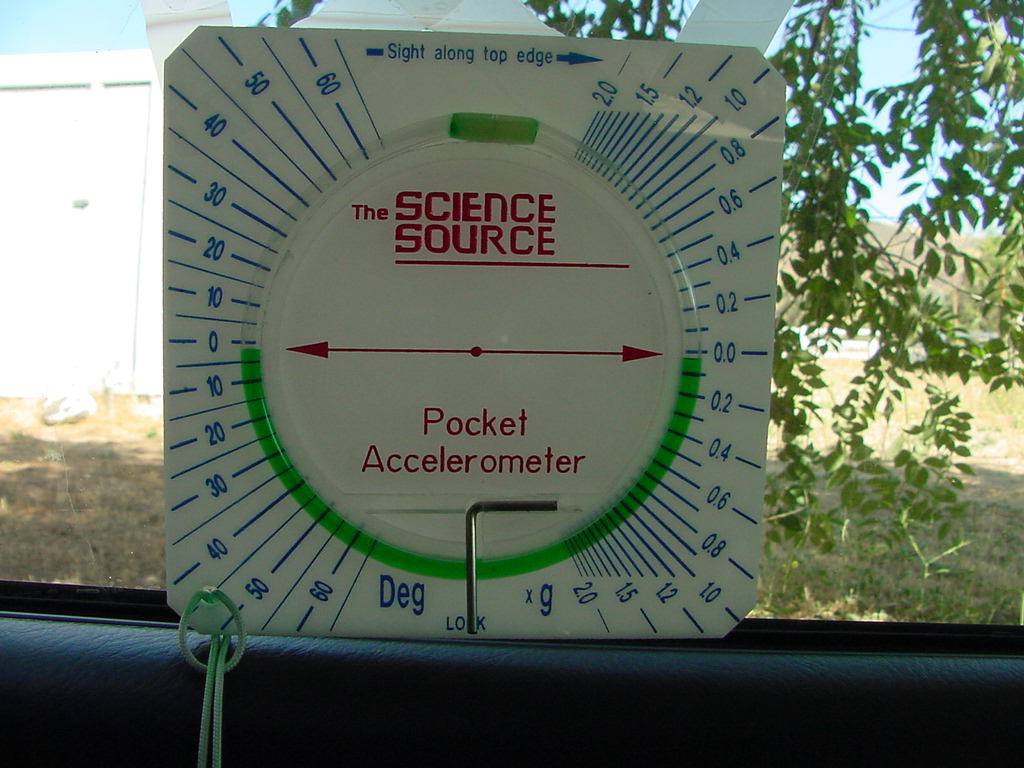Zero acceleration

A glimpse at full acceleration of Honda Civic, about .18g's or 10deg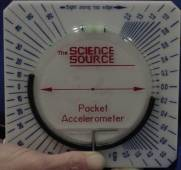10o or .2g's of acceleration

Direction of acceleration to right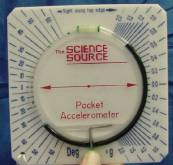45o of acceleration or 1g

Direction of acceleration to left

1. The velocity of an object is the rate of change of its position. As a basis for understanding this concept:

 a. Students know position is defined in relation to some choice of a standard reference point and a set of reference directions. b. Students know that average speed is the total distance traveled divided by the total time elapsed and that the speed of an object along the path traveled can vary. c. Students know how to solve problems involving distance, time, and average speed. d. Students know the velocity of an object must be described by specifying both the direction and the speed of the object. e. Students know changes in velocity may be due to changes in speed, direction, or both.

Materials

Explanation of principles involved

1.      Accelerometer
2.      Clear Tubing Loop with water
4.    Food coloring
5.    A vehicle to drive in.

Acceleration is the change in velocity over time and the accelerometer will allow the students to easily measure the acceleration changes in their car as they drive to school or on another errand.  The tool works by measuring the degree of acceleration in degrees.  The student then would use their knowledge of trigonometry to convert the degrees into gravitational acceleration (g’s) using the formula  a=tan(q) where a is the acceleration in g’s and q is the degree measured with the accelerometer.

Procedure:

1. Take off clear tube and fill it half way with water, add food coloring to color water for easy data reading, recap, put it back on the accelerometer so that both ends of water are on the zero.
2. While sitting in the car, hold the accelerometer with the arrows pointed in the direction of movement of the vehicle.
3. Allow 2-3 seconds for acceleration and then lock the accelerometer and record the units of acceleration in your data table.
4. Record the acceleration every 30 seconds for 5 minutes and record in the data table.  Convert degrees to g’s using the formula a=tan(q).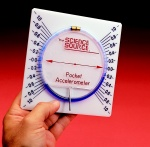This is the pocket accelerometer we will use today.  The zero points are a mark of no acceleration.  Positive forward acceleration is seen when the liquid rises on the right side of the accelerometer, deceleration is recorded when the liquid is below the 0.0 mark on the right hand side

The accelerometer should be held vertically with the arrows facing the direction of motion

To convert degrees to g’s, use the formula

a=tan(q)

Here is a video of what a decelerating car would show up like on the pocket accelerometer. Notice there is a -.2g deceleration.

Here is a short video showing a typical car ride, typical acceleration averaged about 2deg or 0.04g's, with deceleration a little sharper as the car needed to stop for cars in front of it, the deceleration was about 0.1g's.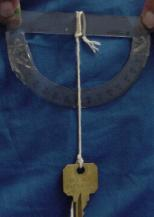If you can’t buy a fancy pocket accelerometer, it is easy to make one with a protractor, string and a weight at the end of the string.  With zero acceleration, the weight hangs vertically at the 90o mark.  During acceleration, measure the angular deflection of the string.  The greater the angle, the greater the acceleration.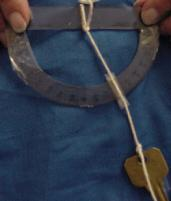In this example, the acceleration is measured at 30o, using the conversion, the acceleration would be 0.58g’s.

 Time(s) Degrees (q) Acceleration g’s (a) 0 30 60 90 120 150 180 210 240 270 300

Use this as your data table